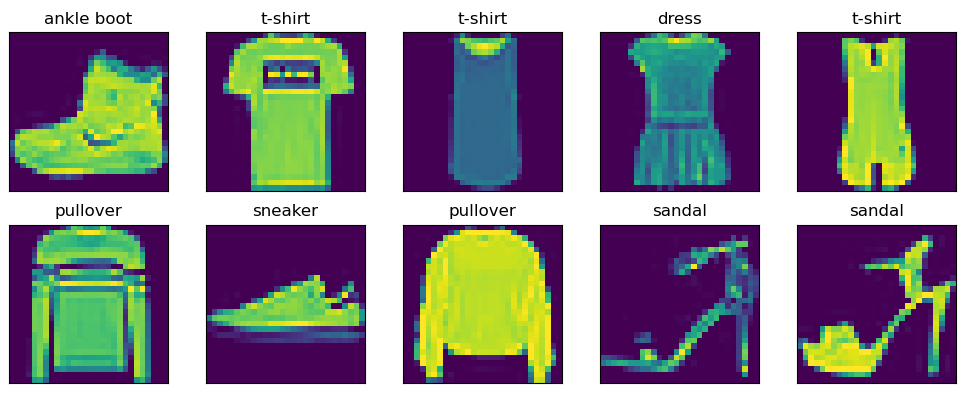# Pytorch之Softmax多分类任务

## 1 数据集

### 1.1 FashionMNISTdef load_dataset():
return mnist_train, mnist_test



### 1.2 构造数据集

start = time.time()
train_iter = torch.utils.data.DataLoader(mnist_test, batch_size=1024, shuffle=True, num_workers=2)
for x_test, y_test in train_iter:
print(x_test.shape)
print('%.2f sec' % (time.time() - start))

#结果
torch.Size([1024, 1, 28, 28])
torch.Size([1024, 1, 28, 28])
torch.Size([1024, 1, 28, 28])
torch.Size([1024, 1, 28, 28])
torch.Size([1024, 1, 28, 28])
torch.Size([1024, 1, 28, 28])
torch.Size([1024, 1, 28, 28])
torch.Size([1024, 1, 28, 28])
torch.Size([1024, 1, 28, 28])
torch.Size([784, 1, 28, 28])
2.60 sec


def make_dataset():
x = torch.linspace(0, 100, 100, dtype=torch.float32).reshape(-1, 2)
y = torch.randn(50 )
dataset = torch.utils.data.TensorDataset(x, y)
return dataset## 2 Softmax多分类

### 2.1 softmax计算实现

def softmax(x):
s = torch.exp(x)
return s / torch.sum(s, dim=1, keepdim=True)# 此处触发了广播机制

a = torch.tensor([[1,2,3.],[2,3,1.]])
print(softmax(a))
#结果：
tensor([[0.0900, 0.2447, 0.6652],
[0.2447, 0.6652, 0.0900]])


### 2.2 交叉熵计算实现

def crossEntropy(logits,y):
c = -torch.log(logits.gather(1,y.reshape(-1,1)))

logits = torch.tensor([[0.1, 0.3, 0.6], [0.5, 0.4, 0.1]])
y = torch.LongTensor([2, 1])
c = crossEntropy(logits,y)
print(c)

#结果
tensor(1.4271)


### 2.3 准确率计算实现

y_true = torch.tensor([[2,1]])
logits = torch.tensor([[0.1,0.3,0.6],[0.5,0.4,0.1]])
y_pred = logits.argmax(1)
print(y_pred)

#结果
tensor([2, 0])


def accuracy(y_true,logits):
acc = (logits.argmax(1) == y_true).float().mean()
return acc.item()

print(accuracy(y_true,logits))
#结果
0.5


### 2.4 评估模型

def evaluate(data_iter, forward, input_nodes, w, b):
acc_sum, n = 0.0, 0
for x, y in data_iter:
logits = forward(x, input_nodes, w, b)
acc_sum += (logits.argmax(1) == y).float().sum().item()
n += len(y)
return acc_sum / n


### 2.5 分类模型实现

w = torch.tensor(np.random.normal(0, 0.5, [input_nodes, output_nodes]),
for epoch in range(epochs):
for i, (x, y) in enumerate(train_iter):
logits = forward(x, input_nodes, w, b)
l = crossEntropy(y, logits)
l.backward()
acc = accuracy(y, logits)
if i % 50 == 0:
print("Epoches[{}/{}]---batch[{}/{}]---acc{:.4}---loss {:.4}".format(
epoches, epoch, len(mnist_train) // batch_size, i, acc,l))
acc = evaluate(test_iter, forward, input_nodes, w, b)
print("Epoches[{}/{}]--acc on test{:.4}".format(epochs, epoch, acc))
# 结果：
Epochs[8000/20]--acc on test0.8323
Epochs[8000/21]---batch[468/0]---acc0.8516---loss 47.13
Epochs[8000/21]---batch[468/50]---acc0.8203---loss 67.22
Epochs[8000/21]---batch[468/100]---acc0.9219---loss 38.74
Epochs[8000/21]---batch[468/150]---acc0.8516---loss 57.39
Epochs[8000/21]---batch[468/200]---acc0.8281---loss 74.76
Epochs[8000/21]---batch[468/250]---acc0.8672---loss 55.32
Epochs[8000/21]---batch[468/300]---acc0.8281---loss 60.19


## 3 总结

### 引用

动手深度学习

示例代码：https://github.com/moon-hotel/DeepLearningWithMe

### 推荐阅读

想明白多分类必须得谈逻辑回归

Pytorch之Linear与MSELoss

Pytorch之拟合正弦函数你会吗？

你告诉我什么是深度学习

11-15441608-137193
09-112万+
09-101万+
09-01167
02-12137
06-063627
04-011119
07-06487
08-309145
11-24164
06-132111
12-0751
05-061516
11-201万+
08-281223
02-1483